# Mean

Quick way of remembering that the mean is the average.

You can calculate the mean by adding up all of the numbers in the set and then dividing by how many numbers there are in the set.

Here are some examples:

#1) What is the average height of the children at the babysitter's house?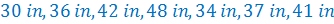Step 1: Add up all of the heights.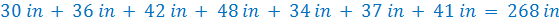Step 2: Divide the sum by the number of heights.

There were 7 heights given. So we will divide by 7.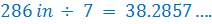Therefore, we can determine that the average height of a child at the babysitter's house is about 32 in.

#2) Deedee forgot to turn in her last assignment. Determine how this will affect her average if her first 9 assignment had grades of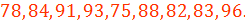Note that this question is not just simply asking for the average or the mean. It is asking how the mean will change when a score of 0 is added in.

First, we will determine the mean of the first nine scores.

Start by adding the scores together.Next, we will divide the total by 9 because there are 9 scores.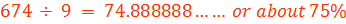Now, we want to compare this average to the average of 10 scores, where the tenth score is 0.

Recall the first 9 scores had a sum of 674. Now we will add on the 10th score.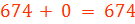Next, we will divide the sum of all ten scores by 10.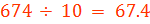Finally, we can compare the two averages.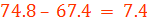If Deedee forgets to turn in the last assignment, her average will drop about 7.4 points.

Let's Review:

To calculate the mean of a set of data you should first add up all the data values together. Then divide by the number of values that you added. So if you have 5 scores, add them up and then divide by 5. If you have 7 grades, add them up and divide by 7. This will give you the mean or the average.